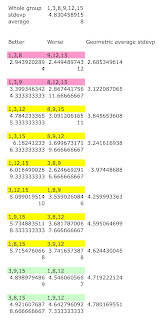## Wednesday, February 2, 2011

### A method to create balanced groups

I like for my students to work together in groups. Unfortunately, they always choose to sit with their friends. So I have to assign their groups.

Let's say on the first day of class, I give them a 20 point quiz to see how much of last year's math they remember. I can also use that information to create balanced groups; I don't want to put all the weak students in one group and all the strong students in another. Rather, I would like each group to include weak students, strong students, and average students.

One way to look at this problem is that I want each group's average to be close to the class average. One could design an algorithm to create the groups this way. But I realized as I was designing it that one has to make ad hoc rules that throw out (a) groups composed of entirely average students and (b) groups composed evenly of strong and weak students but no average ones. I wanted a more elegant algorithm.

The other way to look at the problem is that I want each group to be as diverse as possible. If one maximizes each group's standard deviation, then (a) groups are ruled out. It is true that (b) groups would have the largest standard deviation possible -- but the magic here is that standard deviation is non-linear. Let's say that you have starting forming two groups -- group 1 {S, W, W} and group 2 {S, A, A} -- you have two more students to assign {S, A}. It is true that assigning the strong student to group 1 {S, S, W, W} makes its standard deviation bigger than assigning to it the average student {S, A, W, W}. In other words, std. dev. 1s > std. dev. 1a. But the key is that it makes a much bigger difference to group 2: std. dev. 2s is much, much bigger than std. dev. 2a.

Algebraically, (std. dev. 1s - std. dev. 1a) < (std. dev. 2s - std. dev. 2a). Equivalently, (std. dev. 1s + std. dev. 2a) < (std. dev. 2s + std. dev. 1a). Without algebra, the point is that (b) groups would never happen if you try to maximize the standard deviation of each group -- overall, you're always better to maximize the standard deviation of the least diverse group first. With this fact in mind, this becomes a simple maximization problem. This is an extremely powerful idea: to create the most balanced groupings, you make each group as diverse as possible. Below is an example. A fictional class of six will be divided into two groups.As you can see, each colored line represents a possible set of groupings. The worst groupings are highlighted in red, the mediocre groupings are highlighted in yellow, and the good groupings are highlighted in green. The first cell under a group is its standard deviation, and the second cell is the group's average. I ranked the groupings by creating a geometric average (a b) ^ 0.5 of the two groups' standard deviations. I do generally agree with the rankings. In the red groupings, the groups are skewed: all strong students in one, all weak students in the other. As a result, both groups have low standard deviations. In the yellow groupings, one group is skewed, and the other group includes too many average students. As a result, one group has a high standard deviation, while the other has a low standard deviation. In the green groupings, both groups are balanced, so both have decent standard deviations.

As you'll notice, even for the best groupings, the average standard deviation of the groups doesn't exceed the standard deviation of the entire class. That's as it should be, but I'll leave that proof to an industrious reader.

Another, more realistic (though still fictional) example follows: dividing a 16-student class into four groups. There are too many possible groupings to list (more than 2.6 million), so I used an algorithm to pick a reasonably good solution. (I started by putting the four students in the very middle in different groups, then given that initial condition, the algorithm then assigned the remaining students in a way that attempted to maximize each group's standard deviation.) You can see the results below:I lettered the students from A to P, strongest to weakest, and indicated a percentage grade for each. The four middle students were G, H, I, and J. You can see that the algorithm was able to do a decent job of maximizing each group's standard deviation (its explicit task); some came out slightly ahead, but they're all relatively large. But you can see how well the algorithm did creating balanced groups: 82.2, 82.4, 84, and 84.2. Remember, this result is an accidental byproduct of trying to create a solution that maximizes each group's standard deviation.

Why do I care so much about this? For one, I really do like having an algorithm that creates evenly balanced groups in my class. But two, I want to build this algorithm into a tabulation program -- for partial round robins. In a partial round robin, each team should hit a representative cross-section of the pool. Consider a 12-team round robin with 6 preliminary rounds. It's too tight to run a regular tournament and respect win-loss brackets. But if you can rank the teams before they arrive, then using my algorithm, each team could debate six opponents that are strong, mediocre, and weak.

#### 1 comment:

1.There is a program that does that, no need to build it. I hope it will help: https://www.groupsmaker.com